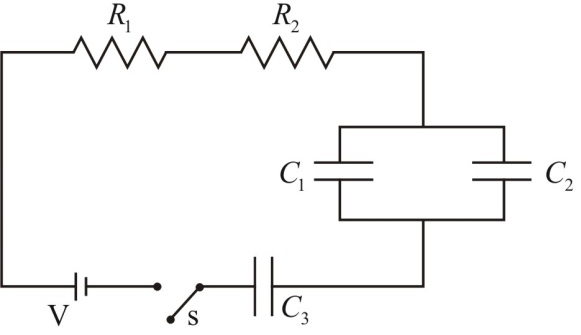# Problem: Consider the circuit shown with R1 = 25 Ω; R2 = 33 Ω;  C1 = 12 μF; C2 = 23 μF; C3 = 46 μF and V = 6.0 VDraw an equivalent circuit with one resistor and one capacitor and label it with the values of the equivalent resistor and capacitor

###### FREE Expert Solution

Equivalent resistance for resistors in series:

$\overline{){{\mathbf{R}}}_{{\mathbf{eq}}}{\mathbf{=}}{{\mathbf{R}}}_{{\mathbf{1}}}{\mathbf{+}}{{\mathbf{R}}}_{{\mathbf{2}}}{\mathbf{+}}{\mathbf{.}}{\mathbf{.}}{\mathbf{.}}{\mathbf{+}}{{\mathbf{R}}}_{{\mathbf{n}}}}$

For capacitors in parallel, the equivalent capacitance is:

$\overline{){{\mathbf{C}}}_{\mathbf{e}\mathbf{q}}{\mathbf{=}}{{\mathbf{C}}}_{{\mathbf{1}}}{\mathbf{+}}{{\mathbf{C}}}_{{\mathbf{2}}}{\mathbf{+}}{\mathbf{.}}{\mathbf{.}}{\mathbf{.}}{\mathbf{+}}{{\mathbf{C}}}_{{\mathbf{n}}}}$

For 2 capacitors in series, the equivalent capacitance is:

$\overline{){{\mathbf{C}}}_{\mathbf{e}\mathbf{q}}{\mathbf{=}}\frac{{\mathbf{C}}_{\mathbf{1}}{\mathbf{C}}_{\mathbf{2}}}{{\mathbf{C}}_{\mathbf{1}}\mathbf{+}{\mathbf{C}}_{\mathbf{2}}}}$

79% (418 ratings)###### Problem Details

Consider the circuit shown with R1 = 25 Ω; R2 = 33 Ω;  C1 = 12 μF; C2 = 23 μF; C3 = 46 μF and V = 6.0 V

Draw an equivalent circuit with one resistor and one capacitor and label it with the values of the equivalent resistor and capacitor